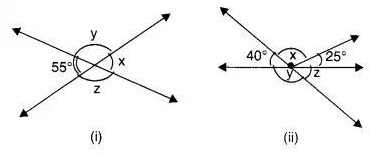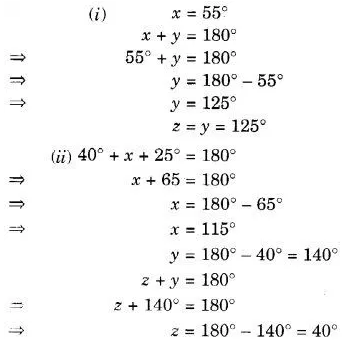# Find the values of the angles x, y and z in each of the following:

Find the values of the angles x, y and z in each of the following: